# Long Run vs. Short Run

• Production function

• The quantity of output a firm produces depends on the quantity of inputs

• This relationship is known as the firm's production function

• Inputs and outputs

• Fixed input is an input whose quantity is fixed for a period of time and cannot be varied (ie. Land)

• Variable input is an input whose quantity can vary over a short period of time (ie. Labor)

• Long run vs. short run

• In the long run, there are no fixed inputs. All costs are variable

• In the short run, at least one input will be fixed

# Marginal Product of Labor (MPL)

• Definition

• change in quantity of output produced by one additional unit of labor
• Formula

•• Graph

• Downward sloping

• Quantity of Labor on the x-axis

• MPL of labor on the y-axis

• Example 1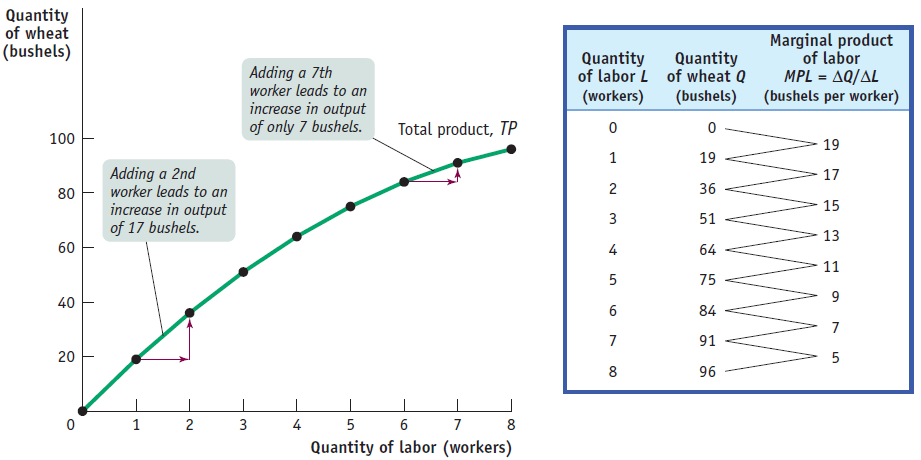• The table shows the production function, the relationship between the quantity of the variable input (labor, measured in number of workers) and the quantity of output (wheat, measured in bushels) for a given quantity of the fixed input.

• It also shows the marginal product of labor on George and Martha's farm.

• The total product curve shows the production function graphically.

• It slopes upward because more wheat is produced as more workers are employed.

• It also becomes flatter because the marginal product of labor declines as more and more workers are employed.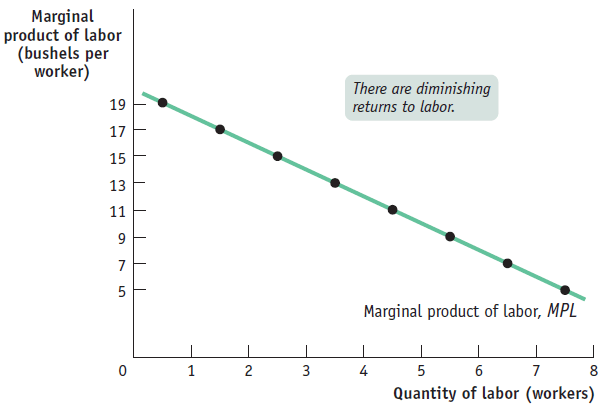• The marginal product of labor curve plots each worker's marginal product, the increase in the quantity of output generated by each additional worker.

• The change in the quantity of output is measured on the vertical axis and the number of workers employed on the horizontal axis.

• The first worker employed generates an increase in output of 19 bushels, the second worker generates an increase of 17 bushels, and so on.

• The curve slopes downward due to the diminishing returns to labor

# Different Types of Marginal Returns

• Increasing marginal returns

• The MPL increases as you hire more workers
• Diminishing marginal returns

• The MPL decreases but the total output increases
• Negative marginal returns

• The MPL decreases as well as the total output
• Graph# Was Thomas Malthus Correct?

• In his book, An Essay On the Principle of Population, Thomas Malthus predicted that, based on the principle of diminishing marginal returns, we would have to brace ourselves for a widespread starvation of the masses.

• Thomas Carlyle coined the phrase "dismal science" - the term has caught on to describe economics as a gloomy subject

• Was Malthus right?

• No, he did not account for the increase in TECHNOLOGY!

# Fixed, Variable and Total Cost

• Fixed cost

• cost that does not depend on the quantity of output produced (ie. franchising fee)
• Variable cost

• cost that depends on the quantity of output produced (ie. bread, cheese, part-time workers)
• Total cost

• Sum of fixed and variable cost

•• Graph• The total cost curve slopes upward because the number of workers employed, and hence total cost, increases as the quantity of output increases.

• The curve gets steeper as output increases due to diminishing returns to labor.

# Average Cost

• Average total cost

• total cost per unit of output

•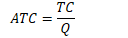• Average fixed cost

• fixed cost per unit of output

•• Average variable cost

• variable cost per unit of output

•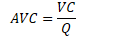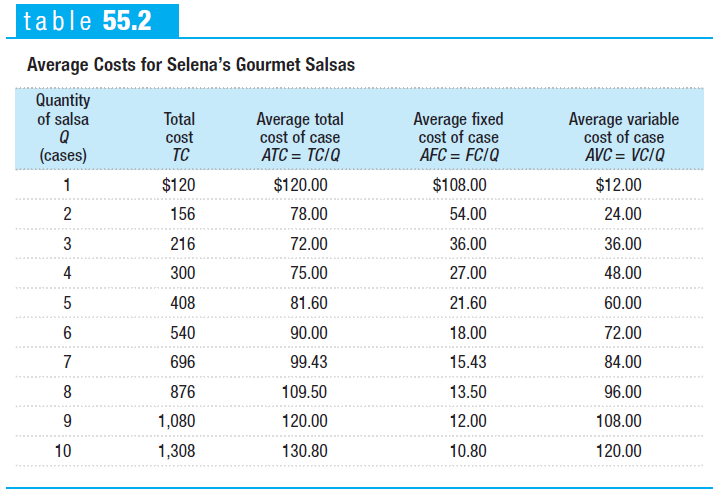# Marginal Cost

• Meaning

• change in total cost generated by one additional unit of output

• change in total cost divided by change in quantity of output

• Formula

•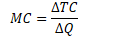# Relationship Between ATC and MC Curves

• At the minimum-cost output, average total cost is equal to marginal cost - ALWAYS!

• At output less than the minimum-cost output, MC is less than ATC and the ATC is rising

• At output greater than the minimum-cost output, MC is greater than ATC and ATC is rising

• Ideal Graph• MC: marginal cost

• ATC: average total cost

• AVC: average variable cost

• AFC: average fixed cost

• Typical Graph• Many firms experience increasing marginal product before diminishing marginal product.

• As a result, they have cost curves shaped like those in this figure.

# True or False Questions

• ATC is always greater than AVC by a constant amount

• Reason: The distance between ATC and AVC is AFC

• If a firm shuts down in the short run, its profits will equal zero

• Reason: Fixed cost is a cost that you will incur even if you shut down

• Equations:

• Total cost = Fixed cost + Variable cost

• Profit = Total revenue - Total cost

• Price vs. average variable cost

• If P > AVC, stay in business

• If P < AVC, then shutdown